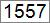Hello Learner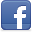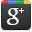Contributor
Stats
 Views : 97256 Comments : 0 Rating : 3
Actions

# Print Perfect Numbers from 1 to 1000 using C# / VB.NET

By Ashok Nalam on 02 Jul 2013 | Category: C# | Tagged: number .NET VB.NET
The program prints perfect numbers between 1-1000 using C#.NET/VB.NET.Add Comment   |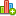Rate this resource  [Rated 3.00/5 by 1 people]

### Introduction

Perfect number is a positive integer that is equal to the sum of its proper positive divisors.  (or)

Perfect number is a number that is half the sum of all of its positive divisors(including itself)

Example:

6 is a  Perfect number.

1, 2, 3 are its proper positive divisors and 1 + 2 + 3 = 6 (or)

1, 2, 3,6 are its positive divisors and (1 + 2 + 3 + 6) / 2 = 6

#### C#.NET Program:

The program prints the Perfect Numbers between 1 - 1000 numbers. If you want to print more perfect numbers, you can increase the condition value (1000) in for loop.
```namespace DotNetMirror
{
class PerfectNumbers1_1000
{
static void Main()
{
Console.WriteLine("/***** Program - Perfect Numbers From 1 to 1000 *****/");
int number, sumOfNumbers = 0, i = 1;
Console.WriteLine("Perfect Numbers From 1 to 1000 are: ");
for (number = 1; number <= 1000; number++)
{
i = 1;
sumOfNumbers = 0;
while (i < number)
{
if (number % i == 0)
sumOfNumbers = sumOfNumbers + i;
i++;
}
if (sumOfNumbers == number)
{
Console.WriteLine("{0} is a Perfect Number", number);
}
}
}
}
}```

#### VB.NET Program:

```Namespace DotNetMirror
Class PerfectNumbers1_1000
Shared Sub Main()
Console.WriteLine("/***** Program - Perfect Numbers From 1 to 1000 *****/")
Dim number As Integer, sumOfNumbers As Integer = 0, i As Integer = 1
Console.WriteLine("Perfect Numbers From 1 to 1000 are: ")
For number = 1 To 1000
i = 1
sumOfNumbers = 0
While i < number
If number Mod i = 0 Then
sumOfNumbers = sumOfNumbers + i
End If
i += 1
End While
If sumOfNumbers = number Then
Console.WriteLine("{0} is a Perfect Number", number)
End If
Next
End Sub
End Class
End Namespace```

#### Output: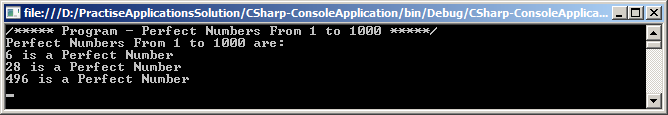Add CommentRate this resource  [Rated 3.00/5 by 1 people]
 Name Email WebSite